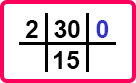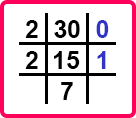# Decimal to Binary, Octal and Hexadecimal Conversion

## Decimal to Binary, Octal, and Hexadecimal Conversion:

In this article, I am going to discuss Decimal to Binary, Octal, and Hexadecimal Conversion with Examples. Please read our previous article where we discussed Number Systems with Examples.

##### Decimal to Binary Conversion:

Let us take a decimal number 30. How do we know that this is in the decimal number system? See 30 can be octal and hexadecimal also. So, to differentiate between these all we will write the number with base,

(30)10

So how much it is equal in binary form? So, for this, the method of conversion is to take this number and go on dividing this by 2 because we are converting it into binary. So let us divide 30 by 2,Here the remainder is 0. We didn’t get any remainder. Now let us divide it further.Here we have the remainder 1. Let us complete the division until the digit becomes less than 2.Now we have to take the remainder (digits) in the bottom to up order.So, the digits are 11110. This is the binary form of 30. So,

(30)10 = (11110)2

Let us take one more number 53 in the decimal number system. Let us convert this into binary.We have divided 54 by 2 and we get the remainder of 0.We have just followed the previous steps and noted the remaining digits. So, the digits are 110110.

(54)10 = (110110)2

That’s it. This is how we can convert a decimal number to a binary number. We will see the conversion of binary to decimal in the next article. In this article, we will see decimal to binary, octal, and hexadecimal. We have seen decimal to binary so let us see decimal to octal.

##### Decimal to Octal Conversion:

Let us take 30 in decimal and convert it into octal. See the procedure is the same but here we have to divide the number by 8. Let us see the division.We have divided 30 by 8 and we get the remainder of 6. But the number 3 cannot divide by 8 so we have left the division at 3. So, the digits are 36.

(30)10 = (36)8

So decimal number 30 is equal to 36 in the octal number system. Now let us take 54. Again, we divide 54 by 8,The digits are 66.

(54)10 = (66)8

54 in the decimal system is equivalent to 66 in the octal system. Now let us convert the decimal system to a hexadecimal system.

Let us take 30 in the decimal system. Here we have to divide the decimal number by 16.We get 1 and 14 digits. Here 1 will remain the same but we know that 14 is written as ‘E’ in the hexadecimal system. So, 1 and 14 will be written as 1E in hexadecimal.

(30)10 = (1E)16

Here we have written base as 16. Now let us convert 54 into a hexadecimal system.The digits are 3 and 6. So,

(54)10 = (36)16

So that’s it. See the approach is the same for converting a decimal number system to a binary, octal, and hexadecimal number system. For all conversions, we have to divide that number. And note down the remainders in the bottom to up order.